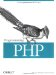# 3.4 Function Parameters

Functions can expect, by declaring them in the function definition, an arbitrary number of arguments.

There are two different ways of passing parameters to a function. The first, and more common, is by value. The other is by reference.

#### 3.4.1 Passing Parameters by Value

In most cases, you pass parameters by value. The argument is any valid expression. That expression is evaluated, and the resulting value is assigned to the appropriate variable in the function. In all of the examples so far, we've been passing arguments by value.

#### 3.4.2 Passing Parameters by Reference

Passing by reference allows you to override the normal scoping rules and give a function direct access to a variable. To be passed by reference, the argument must be a variable; you indicate that a particular argument of a function will be passed by reference by preceding the variable name in the parameter list with an ampersand (&). Example 3-5 revisits our doubler( ) function with a slight change.

##### Example 3-5. Doubler redux
`function doubler(&\$value) {   \$value = \$value << 1; } \$a = 3; doubler(\$a); echo \$a;`

Because the function's \$value parameter is passed by reference, the actual value of \$a, rather than a copy of that value, is modified by the function. Before, we had to return the doubled value, but now we change the caller's variable to be the doubled value.

Here's another place where a function contains side effects: since we passed the variable \$a into doubler( ) by reference, the value of \$a is at the mercy of the function. In this case, doubler( ) assigns a new value to it.

A parameter that is declared as being passed by reference can only be a variable. Thus, if we included the statement <?= doubler(7); ?> in the previous example, it would issue an error.

Even in cases where your function does affect the given value, you may want a parameter to be passed by reference. When passing by value, PHP must copy the value. Particularly for large strings and objects, this can be an expensive operation. Passing by reference removes the need to copy the value.

#### 3.4.3 Default Parameters

Sometimes, a function may need to accept a particular parameter in some cases. For example, when you call a function to get the preferences for a site, the function may take in a parameter with the name of the preference to retrieve. If you want to retrieve all the preferences, rather than using some special keyword, you can just not supply an argument. This behavior works by using default arguments.

To specify a default parameter, assign the parameter value in the function declaration. The value assigned to a parameter as a default value cannot be a complex expression; it can only be a constant.

`function get_preferences(\$which_preference = "all" ) {     // if \$which_preference is "all", return all prefs;     // otherwise, get the specific preference requested... }`

When you call get_preferences( ), you can choose to supply an argument. If you do, it returns the preference matching the string you give it; if not, it returns all preferences.

A function may have any number of parameters with default values. However, they must be listed after all the parameters that do not have default values.

#### 3.4.4 Variable Parameters

A function may require a variable number of arguments. For example, the get_preferences( ) example in the previous section might return the preferences for any number of names, rather than for just one. To declare a function with a variable number of arguments, leave out the parameter block entirely.

`function get_preferences(  ) {   // some code }`

PHP provides three functions you can use in the function to retrieve the parameters passed to it. func_get_args( ) returns an array of all parameters provided to the function, func_num_args( ) returns the number of parameters provided to the function, and func_get_arg( ) returns a specific argument from the parameters.

`\$array = func_get_args(  ); \$count = func_num_args(  ); \$value = func_get_arg(argument_number);`

In Example 3-6, the count_list( ) function takes in any number of arguments. It loops over those arguments and returns the total of all the values. If no parameters are given, it returns false.

##### Example 3-6. Argument counter
`function count_list(  ) {   if(func_num_args(  ) == 0) {     return false;   }   else {     for(\$i = 0; \$i < func_num_args(  ); \$i++) {       \$count += func_get_arg(\$i);     }     return \$count;   } } echo count_list(1, 5, 9);`

The result of any of these functions cannot directly be used as a parameter to another function. To use the result of one of these functions as a parameter, you must first set a variable to the result of the function, then use that in the function call. The following expression will not work:

`foo(func_num_args(  ));`

`\$count = func_num_args(  ); foo(\$count);`

#### 3.4.5 Missing Parameters

PHP lets you be as lazy as you want when you call a function, you can pass any number of arguments to the function. Any parameters the function expects that are not passed to it remain unset, and a warning is issued for each of them:

`function takes_two( \$a, \$b ) {   if (isset(\$a)) { echo " a is set\n"; }   if (isset(\$b)) { echo " b is set\n"; } } echo "With two arguments:\n"; takes_two(1, 2); echo "With one argument:\n"; takes_two(1); With two arguments:  a is set  b is set With one argument: Warning:  Missing argument 2 for takes_two(  )  in /path/to/script.php on line 6 a is set`Programming PHP
ISBN: 1565926102
EAN: 2147483647
Year: 2007
Pages: 168

Similar book on Amazon